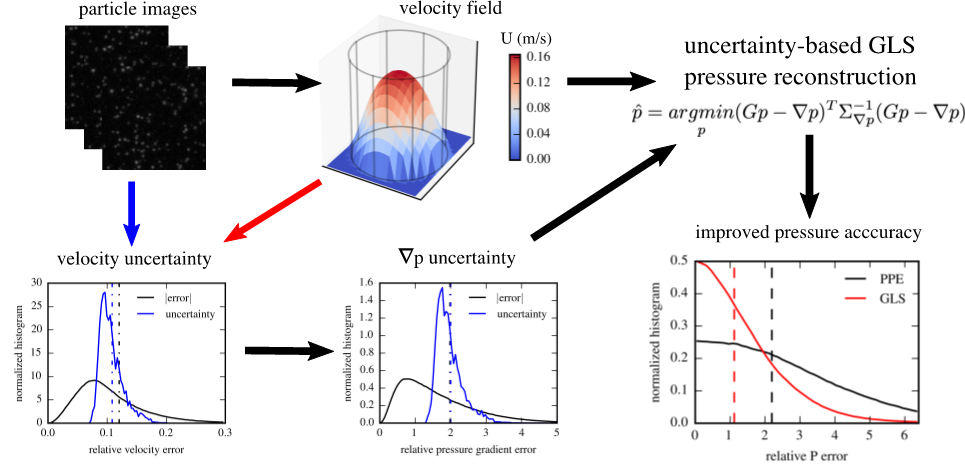Using uncertainty to improve pressure field reconstruction from PIV/PTV flow measurements
16191## 17 Jul Using uncertainty to improve pressure field reconstruction from PIV/PTV flow measurements

Measurement of pressure in a fluid flow is essential in engineering applications as well as in investigations of flow physics. Pressure measurement devices such as wall pressure ports, static tubes, pressure-sensitive paint, etc., can be invasive, and provide only point measurements or a surface distribution. Further, most pressure measurement techniques have limitations in dynamic range and resolvable frequency bandwidth. With the development of flow measurement techniques such as particle image velocimetry (PIV) and particle tracking velocimetry (PTV), the velocity fields can be obtained and utilized for instantaneous pressure evaluation. However, the accuracy of the reconstructed pressure fields is significantly affected by the measurement error in the velocity fields.Fig. 1 The instantaneous pressure reconstruction from PIV/PTV data with generalized least-squares (GLS). The velocity uncertainty is propagated to estimate the uncertainty in pressure gradients, which is incorporated in the pressure integration with GLS. Better pressure accuracy can be obtained by GLS compared to the baseline method of solving the pressure Poisson equation (PPE).

In this work, a robust pressure reconstruction method is proposed, which employs the velocity uncertainty to improve the accuracy of reconstructed pressure fields. First, the pressure gradient fields are calculated from velocity fields, while the local and instantaneous pressure gradient uncertainty is estimated from the velocity uncertainty using a linear-transformation-based algorithm. The pressure field is then reconstructed by solving an overdetermined linear system which involves the pressure gradients and boundary conditions. This linear system is solved with generalized least-squares (GLS), which incorporates the previously estimated variances and covariances of the pressure gradient errors as inverse weights to optimize the reconstructed pressure field. The method was validated with 2D synthetic velocity fields. The pressure error reduction by GLS was 50%, with 9.6% velocity errors and 250% with 32.1% velocity errors compared to the existing baseline method of solving the pressure Poisson equation (PPE). The technique was also applied to 3D flow-data from PTV, and the reconstructed pressure fields were in good agreement with the analytical and empirical estimations.Fig. 2: For the pressure reconstruction from the PTV flow-data of a transitional pipe flow: (a) The in-plane velocity (vectors) and out-of-plane vorticity (color scale) on the X-Y plane. (b) The normalized pressure field from GLS with the local minimum pressure predicted around the vortex cores. (c) The pressure drop along x-direction predicted by GLS is in better agreement with the empirical estimation compared to PPE.